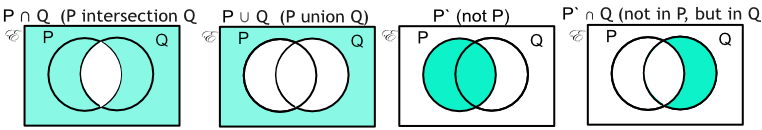Venn Diagrams

# Venn Diagrams

GCSE(F) GCSE(H)

Venn Diagrams are used to display set notation. A Venn diagram consists of one or more circles within a rectangle. The rectangle defines the Universal Set, and each circle is a different set within the Universal Set. Elements not in a set are shown within the rectangle.

If two dice are thrown and added together:Venn Diagrams can be used to determine the interaction of sets:## Examples

1. Given a set of integers from 1 to 15, set A = {multiples of two} and set B = {multiples of three}. The Venn Diagram has been started. What numbers go in the part of the diagram labelled x?Answer: A nn B = {6, 12}

A={2,4,6,8,10,12,14} and B={3,6,9,12,15}

Part x is A overlapping B A nn B = {6, 12}.

2. For the Venn Diagram, above, what numbers go into the part of the diagram marked y?

Answer: A nn B^c = {2, 4, 8, 10, 14}

A={2,4,6,8,10,12,14} and B^c={1,2,4,5,7,8,10,11,13,14}

Elements in A and not in B: A nn B^c = {2, 4, 8, 10, 14}